# Prediction Intervals from merMod Objects

## Introduction

Fitting (generalized) linear mixed models, (G)LMM, to very large data sets is becoming increasingly easy, but understanding and communicating the uncertainty inherent in those models is not. As the documentation for lme4::predict.merMod() notes:

There is no option for computing standard errors of predictions because it is difficult to define an efficient method that incorporates uncertainty in the variance parameters; we recommend lme4::bootMer() for this task.

We agree that, short of a fully Bayesian analysis, bootstrapping is the gold-standard for deriving a prediction interval predictions from a (G)LMM, but the time required to obtain even a respectable number of replications from bootMer() quickly becomes prohibitive when the initial model fit is on the order of hours instead of seconds. The only other alternative we have identified for these situations is to use the arm::sim() function to simulate values. Unfortunately, this only takes variation of the fixed coefficients and residuals into account, and assumes the conditional modes of the random effects are fixed.

We developed the predictInterval() function to incorporate the variation in the conditional modes of the random effects (CMRE, a.k.a. BLUPs in the LMM case) into calculating prediction intervals. Ignoring the variance in the CMRE results in overly confident estimates of predicted values and in cases where the precision of the grouping term varies across levels of grouping terms, creates the illusion of difference where none may exist. The importance of accounting for this variance comes into play sharply when comparing the predictions of different models across observations.

We take the warning from lme4::predict.merMod() seriously, but view this method as a decent first approximation the full bootstrap analysis for (G)LMMs fit to very large data sets.

## Conceptual description

In order to generate a proper prediction interval, a prediction must account for three sources of uncertainty in mixed models:

1. the residual (observation-level) variance,
2. the uncertainty in the fixed coefficients, and
3. the uncertainty in the variance parameters for the grouping factors.

A fourth, uncertainty about the data, is beyond the scope of any prediction method.

As we mentioned above, the arm:sim() function incorporates the first two sources of variation but not the third , while bootstrapping using lme4::bootMer() does incorporate all three sources of uncertainty because it re-estimates the model using random samples of the data.

When inference about the values of the CMREs is of interest, it would be nice to incorporate some degree of uncertainty in those estimates when comparing observations across groups. predictInterval() does this by drawing values of the CMREs from the conditional variance-covariance matrix of the random affects accessible from lme4::ranef(model, condVar=TRUE). Thus, predictInterval() incorporates all of the uncertainty from sources one and two, and part of the variance from source 3, but the variance parameters themselves are treated as fixed.

To do this, predictInterval() takes an estimated model of class merMod and, like predict(), a data.frame upon which to make those predictions and:

1. extracts the fixed and random coefficients
2. takes n draws from the multivariate normal distribution of the fixed and random coefficients (separately)
3. calculates the linear predictor for each row in newdata based on these draws, and
4. optionally incorporates the residual variation (per the arm::sim() function), and,
5. returns newdata with the lower and upper limits of the prediction interval and the mean or median of the simulated predictions

Currently, the supported model types are linear mixed models and mixed logistic regression models.

The prediction data set can include levels that are not in the estimation model frame. The prediction intervals for such observations only incorporate uncertainty from fixed coefficient estimates and the residual level of variation.

## Comparison to existing methods

What do the differences between predictInterval() and the other methods for constructing prediction intervals mean in practice? We would expect to see predictInterval() to produce prediction intervals that are wider than all methods except for the bootMer() method. We would also hope that the prediction point estimate from other methods falls within the prediction interval produced by predictInterval(). Ideally, the predicted point estimate produced by predictInterval() would fall close to that produced by bootMer().

This section compares the results of predictInterval() with those obtained using arm::sim() and lme4::bootMer() using the sleepstudy data from lme4. These data contain reaction time observations for 10 days on 18 subjects.
The data are sorted such that the first 10 observations are days one through ten for subject 1, the next 10 are days one through ten for subject 2 and so on. The example model that we are estimating below estimates random intercepts and a random slope for the number of days.

### Step 1: Estimating the model and using predictInterval()

First, we will load the required packages and data and estimate the model:

set.seed(271828)
data(sleepstudy)
fm1 <- lmer(Reaction ~ Days + (Days|Subject), data=sleepstudy)
display(fm1)
#> lmer(formula = Reaction ~ Days + (Days | Subject), data = sleepstudy)
#>             coef.est coef.se
#> (Intercept) 251.41     6.82
#> Days         10.47     1.55
#>
#> Error terms:
#>  Groups   Name        Std.Dev. Corr
#>  Subject  (Intercept) 24.74
#>           Days         5.92    0.07
#>  Residual             25.59
#> ---
#> number of obs: 180, groups: Subject, 18
#> AIC = 1755.6, DIC = 1760.3
#> deviance = 1751.9

Then, calculate prediction intervals using predictInterval(). The predictInterval function has a number of user configurable options. In this example, we use the original data sleepstudy as the newdata. We pass the function the fm1 model we fit above. We also choose a 95% interval with level = 0.95, though we could choose a less conservative prediction interval. We make 1,000 simulations for each observation n.sims = 1000. We set the point estimate to be the median of the simulated values, instead of the mean. We ask for the linear predictor back, if we fit a logistic regression, we could have asked instead for our predictions on the probability scale instead. Finally, we indicate that we want the predictions to incorporate the residual variance from the model – an option only available for lmerMod objects.

PI.time <- system.time(
PI <- predictInterval(merMod = fm1, newdata = sleepstudy,
level = 0.95, n.sims = 1000,
stat = "median", type="linear.prediction",
include.resid.var = TRUE)
)

Here is the first few rows of the object PI:

fit upr lwr
251.6685 311.3171 196.4096
271.4802 330.9196 214.2175
292.6809 350.9868 237.7714
311.6967 369.2911 254.2238
331.8318 389.7439 278.1857
350.7450 408.1386 294.8506

The three columns are the median (fit) and limits of the 95% prediction interval (upr and lwr) because we set level=0.95. The following figure displays the output graphically for the first 30 observations.

library(ggplot2);
ggplot(aes(x=1:30, y=fit, ymin=lwr, ymax=upr), data=PI[1:30,]) +
geom_point() +
geom_linerange() +
labs(x="Index", y="Prediction w/ 95% PI") + theme_bw()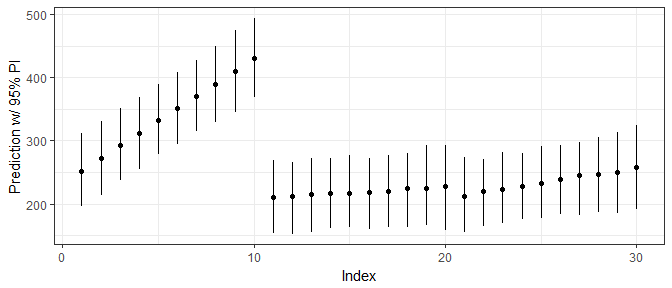### Step 2: Comparison with arm::sim()

How does the output above compare to what we could get from arm::sim()?

PI.arm.time <- system.time(
PI.arm.sims <- arm::sim(fm1, 1000)
)

PI.arm <- data.frame(
fit=apply(fitted(PI.arm.sims, fm1), 1, function(x) quantile(x, 0.500)),
upr=apply(fitted(PI.arm.sims, fm1), 1, function(x) quantile(x, 0.975)),
lwr=apply(fitted(PI.arm.sims, fm1), 1, function(x) quantile(x, 0.025))
)

comp.data <- rbind(data.frame(Predict.Method="predictInterval()", x=(1:nrow(PI))-0.1, PI),
data.frame(Predict.Method="arm::sim()", x=(1:nrow(PI.arm))+0.1, PI.arm))

ggplot(aes(x=x, y=fit, ymin=lwr, ymax=upr, color=Predict.Method), data=comp.data[c(1:30,181:210),]) +
geom_point() +
geom_linerange() +
labs(x="Index", y="Prediction w/ 95% PI") +
theme_bw() +  theme(legend.position="bottom") +
scale_color_brewer(type = "qual", palette = 2)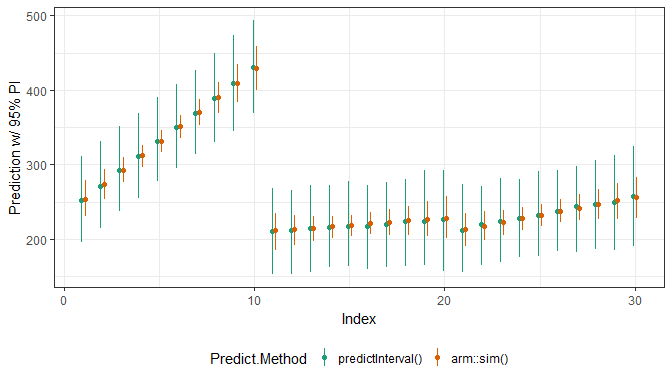The prediction intervals from arm:sim() are much smaller and the random slope for days vary more than they do for predictInterval. Both results are as expected, given the small number of subjects and observations per subject in these data. Because predictInterval() is incorporating uncertainty in the CMFEs (but not the variance parameters of the random coefficients themselves), the Days slopes are closer to the overall or pooled regression slope.

### Step 3: Comparison with lme4::bootMer()

As quoted above, the developers of lme4 suggest that users interested in uncertainty estimates around their predictions use lme4::bootmer() to calculate them. The documentation for lme4::bootMer() goes on to describe three implemented flavors of bootstrapped estimates:

1. parametrically resampling both the “spherical” random effects u and the i.i.d. errors $$\epsilon$$
2. treating the random effects as fixed and parametrically resampling the i.i.d. errors
3. treating the random effects as fixed and semi-parametrically resampling the i.i.d. errors from the distribution of residuals.

We will compare the results from predictInterval() with each method, in turn.

#### Step 3a: lme4::bootMer() method 1

##Functions for bootMer() and objects
####Return predicted values from bootstrap
mySumm <- function(.) {
predict(., newdata=sleepstudy, re.form=NULL)
}
####Collapse bootstrap into median, 95% PI
sumBoot <- function(merBoot) {
return(
data.frame(fit = apply(merBoot$t, 2, function(x) as.numeric(quantile(x, probs=.5, na.rm=TRUE))), lwr = apply(merBoot$t, 2, function(x) as.numeric(quantile(x, probs=.025, na.rm=TRUE))),
upr = apply(merBoot\$t, 2, function(x) as.numeric(quantile(x, probs=.975, na.rm=TRUE)))
)
)
}

##lme4::bootMer() method 1
PI.boot1.time <- system.time(
boot1 <- lme4::bootMer(fm1, mySumm, nsim=1000, use.u=FALSE, type="parametric")
)

PI.boot1 <- sumBoot(boot1)

comp.data <- rbind(data.frame(Predict.Method="predictInterval()", x=(1:nrow(PI))-0.1, PI),
data.frame(Predict.Method="lme4::bootMer() - Method 1", x=(1:nrow(PI.boot1))+0.1, PI.boot1))

ggplot(aes(x=x, y=fit, ymin=lwr, ymax=upr, color=Predict.Method), data=comp.data[c(1:30,181:210),]) +
geom_point() +
geom_linerange() +
labs(x="Index", y="Prediction w/ 95% PI") +
theme_bw() +  theme(legend.position="bottom") +
scale_color_brewer(type = "qual", palette = 2)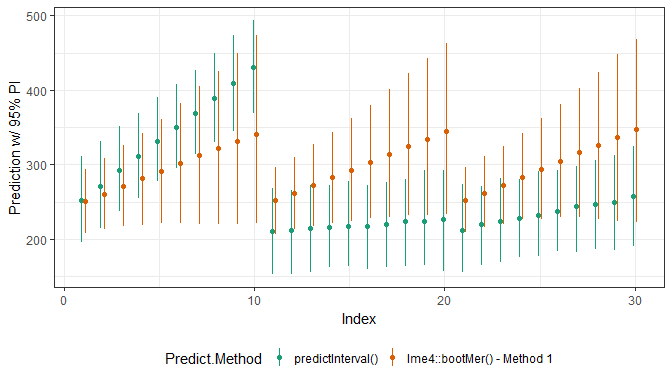The intervals produced by predictInterval, represented in green, cover the point estimates produced by bootMer in every case for these 30 observations. Additionally, in almost every case, the predictInterval encompasses the entire interval presented by bootMer. Here, the estimates produced by bootMer are re-estimating the group terms, but by refitting the model, they are also taking into account the conditional variance of these terms, or theta, and provide tighter prediction intervals than the predictInterval method.

#### Step 3b: lme4::bootMer() method 2

##lme4::bootMer() method 2
PI.boot2.time <- system.time(
boot2 <- lme4::bootMer(fm1, mySumm, nsim=1000, use.u=TRUE, type="parametric")
)

PI.boot2 <- sumBoot(boot2)

comp.data <- rbind(data.frame(Predict.Method="predictInterval()", x=(1:nrow(PI))-0.1, PI),
data.frame(Predict.Method="lme4::bootMer() - Method 2", x=(1:nrow(PI.boot2))+0.1, PI.boot2))

ggplot(aes(x=x, y=fit, ymin=lwr, ymax=upr, color=Predict.Method), data=comp.data[c(1:30,181:210),]) +
geom_point() +
geom_linerange() +
labs(x="Index", y="Prediction w/ 95% PI") +
theme_bw() +  theme(legend.position="bottom") +
scale_color_brewer(type = "qual", palette = 2)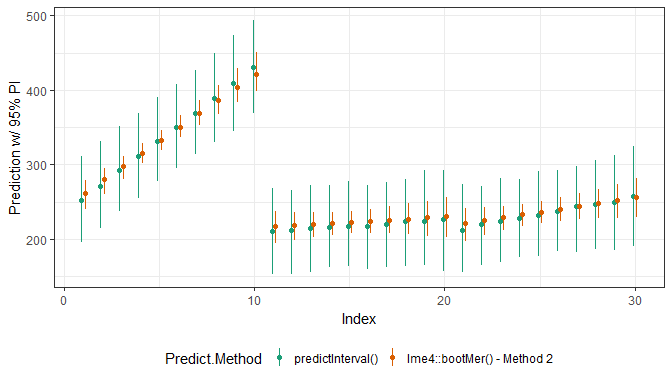Here, the results for predictInterval in green again encompass the results from bootMer, but are much wider. The bootMer estimates are ignoring the variance in the group effects, and as such, are only incorporating the residual variance and the variance in the fixed effects – similar to the arm::sim() function.

#### Step 3c: lme4::bootMer() method 3

##lme4::bootMer() method 3
PI.boot3.time <- system.time(
boot3 <- lme4::bootMer(fm1, mySumm, nsim=1000, use.u=TRUE, type="semiparametric")
)

PI.boot3 <- sumBoot(boot3)

comp.data <- rbind(data.frame(Predict.Method="predictInterval()", x=(1:nrow(PI))-0.1, PI),
data.frame(Predict.Method="lme4::bootMer() - Method 3", x=(1:nrow(PI.boot3))+0.1, PI.boot3))

ggplot(aes(x=x, y=fit, ymin=lwr, ymax=upr, color=Predict.Method), data=comp.data[c(1:30,181:210),]) +
geom_point() +
geom_linerange() +
labs(x="Index", y="Prediction w/ 95% PI") +
theme_bw() +  theme(legend.position="bottom") +
scale_color_brewer(type = "qual", palette = 2)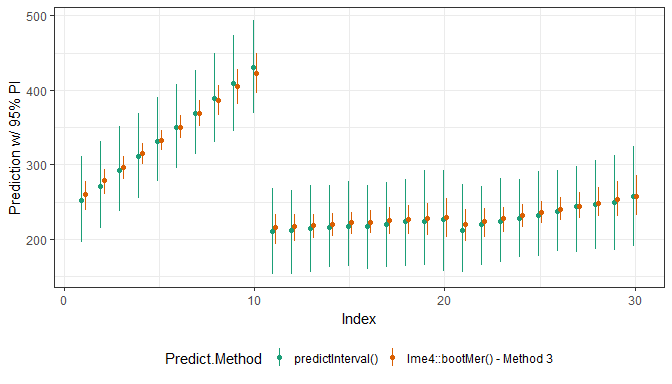These results are virtually identical to those above.

#### Step 3c: Comparison to rstanarm

PI.time.stan <- system.time({
fm_stan <- stan_lmer(Reaction ~ Days + (Days|Subject), data = sleepstudy,
verbose = FALSE, open_progress = FALSE, refresh = -1,
show_messages=FALSE)
zed <- posterior_predict(fm_stan)
PI.stan <- cbind(apply(zed, 2, median), central_intervals(zed, prob=0.95))
})
#>
#>  Elapsed Time: 22.473 seconds (Warm-up)
#>                6.688 seconds (Sampling)
#>                29.161 seconds (Total)
#>
#>
#>  Elapsed Time: 18.132 seconds (Warm-up)
#>                6.679 seconds (Sampling)
#>                24.811 seconds (Total)
#>
#>
#>  Elapsed Time: 13.303 seconds (Warm-up)
#>                6.395 seconds (Sampling)
#>                19.698 seconds (Total)
#>
#>
#>  Elapsed Time: 12.978 seconds (Warm-up)
#>                6.53 seconds (Sampling)
#>                19.508 seconds (Total)

print(fm_stan)
#> stan_lmer(formula = Reaction ~ Days + (Days | Subject), data = sleepstudy,
#>     verbose = FALSE, open_progress = FALSE, refresh = -1, show_messages = FALSE)
#>
#> Estimates:
#> (Intercept) 251.3    6.2
#> Days         10.5    1.7
#> sigma        25.8    1.5
#>
#> Error terms:
#>  Groups   Name        Std.Dev. Corr
#>  Subject  (Intercept) 24.1
#>           Days         6.9     0.08
#>  Residual             25.8
#> Num. levels: Subject 18
#>
#> Sample avg. posterior predictive
#> distribution of y (X = xbar):
#> mean_PPD 298.5    2.6
#>
#> Observations: 180  Number of unconstrained parameters: 45

PI.stan <- as.data.frame(PI.stan)
names(PI.stan) <- c("fit", "lwr", "upr")
PI.stan <- PI.stan[, c("fit", "upr", "lwr")]
comp.data <- rbind(data.frame(Predict.Method="predictInterval()", x=(1:nrow(PI))-0.1, PI),
data.frame(Predict.Method="rstanArm", x=(1:nrow(PI.stan))+0.1, PI.stan))

ggplot(aes(x=x, y=fit, ymin=lwr, ymax=upr, color=Predict.Method), data=comp.data[c(1:30,181:210),]) +
geom_point() +
geom_linerange() +
labs(x="Index", y="Prediction w/ 95% PI") +
theme_bw() +  theme(legend.position="bottom") +
scale_color_brewer(type = "qual", palette = 2)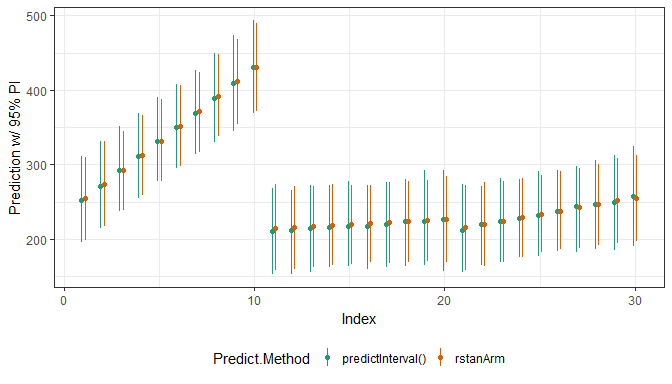### Computation time

Our initial motivation for writing this function was to develop a method for incorporating uncertainty in the CMFEs for mixed models estimated on very large samples. Even for models with only modest degrees of complexity, using lme4::bootMer() quickly becomes time prohibitive because it involves re-estimating the model for each simulation. We have seen how each alternative compares to predictInterval() substantively, but how do they compare in terms of computational time? The table below lists the output of system.time() for all five methods for calculating prediction intervals for merMod objects.

user.self sys.self elapsed
predictInterval() 0.67 0.02 0.68
arm::sim() 1.34 0.00 1.36
lme4::bootMer()-Method 1 46.08 0.04 46.89
lme4::bootMer()-Method 2 47.05 0.03 47.94
lme4::bootMer()-Method 3 46.39 0.02 46.71
rstanarm:predict 95.72 0.03 96.13

For this simple example, we see that arm::sim() is the fastest–nearly five times faster than predictInterval(). However, predictInterval() is nearly six times faster than any of the bootstrapping options via lme4::bootMer. This may not seem like a lot, but consider that the computational time for required for bootstrapping is roughly proportional to the number of bootstrapped simulations requested … predictInterval() is not because it is just a series of draws from various multivariate normal distributions, so the time ratios in the table below represents the lowest bound of the computation time ratio of bootstrapping to predictInterval().

TBC.